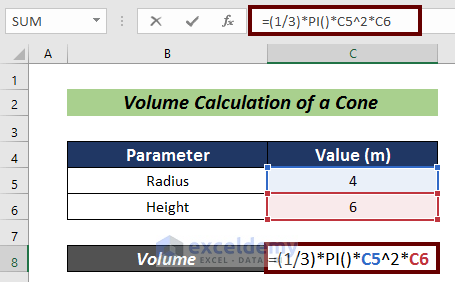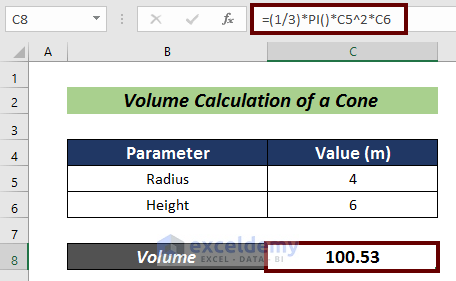# How to Calculate Volume in Excel (7 Different Ways)

Get FREE Advanced Excel Exercises with Solutions!

Volume is a factor related to every matter. We need to have the knowledge of volume of a matter in our day-to-day life. In this article, We are going to learn on how to calculate volume in Excel for 7 different shapes of matter.

## What Is Volume?

Volume is a quantity basically related to physics. It is actually a scalar quantity that symbolizes the amount of space occupied by any three-dimensional substance.

## How to Calculate Volume in Excel: 7 Different Ways

### 1. Volume Calculation of a Sphere

A sphere is basically a solid round figure. We can calculate the volume of a sphere using the following formula:

Volume of a Sphere =  4/3 * Π * r^3

Where, r = Radius of the sphere

Steps:

• Find the related parameters. In this case, we only need to know the radius of the sphere.
• Select a cell for the volume calculation (i.e. C7).• Now, input the following formula in cell C7:
`=(4/3)*PI()*C5^3`

Here, C5 represents the radius of the sphere in meters.• Press ENTER to have the volume of the sphere in m3. If your original data be in another unit then this unit will be changed accordingly.You can practice here for expertise (you will find this portion at the right side of the sheet).Read More: How to Calculate Column Volume in Excel

### 2. Volume Calculation of a Rectangular Solid

A rectangle is a parallelogram all of whose angles are right angles and the adjacent sides are unequal in length. The formula to calculate the volume of a rectangle is as follows:

Volume of a Rectangle= l * b * c

Where,

l = Length of a rectangle
b = Breadth of a rectangle
c = Height of a rectangle

Steps:

• Find the related parameters. Here, we need the length, breadth, and height of the rectangle..
• Choose a cell for the volume calculation (i.e. C9).• Insert the following formula:
`=C5*C6*C7`

Where,

C5 = Length of the rectangle in meters
C6 = Breadth of the rectangle in meters
C7 = Height of the rectangle in meters• Now, hit ENTER and we have the volume of the rectangle in m3.Try yourself in the following section.### 3. Volume Calculation of a Cube

A cube is a parallelogram all of whose angles are right angles and all the sides are equal in length.

Volume of a Cubee= a^3

Where,
a = Length of the sides

Steps:

• Collect the edge length data of a cube..
• Pick a cell for the calculation (i.e. C7).• Write down the formula mentioned below:
`=C5^3`

Where,

C5 = Edge Length in meters• Finally, press ENTER to finish the process in the m3You can practice here by yourself.### 4. Volume Calculation of a Cylinder

A cylinder is actually a solid geometrical figure with a circular or oval cross-section and straight parallel sides.

Volume of a Cylinder = Π * r^2 * h

Where,
r = Radius of the Cylinder
h = Height of the Cylinder

Steps:

• Find the radius and height of a cylinder..
• Now, pick a cell for the volume calculation (i.e. C8).• Next, input the following formula:
`=PI()*C5^2*C6`

Where,
C5 = Radius of the Cylinder in meters
C6 = Height of the Cylinder in meters• Hit ENTER to finish the calculation in m3.For the betterment, you can practice here by yourself.

### 5. Volume Calculation of a Cone

Cone is a solid or hollow object which has a circular base and an apex.

Volume of a Cone =  1/3 * Π * r^2 * h

Where,
r = Radius of the Cone
h = Height of the Cone

Steps:

• Firstly, find the radius and height of a cone.
• Next, choose a cell for the volume calculation (i.e. C8).• Now, input the formula mentioned below:
`=(1/3)*PI()*C5^2*C6`

Where,
C5 = Radius of the Cone in meters
C6 = Height of the Cone in meters

###• Now, press ENTER to have the result in m3.

###Try yourself in the following section.

### 6. Volume Calculation of a Torus

Torus is a large convex molding with a semicircular cross-section.

Volume of a Torus = Π * r^2 * 2 * Π * R

Where,
r = Inner Radius of the Torus
R = Outer Radius of the Torus

Steps:

• Firstly, find the inner and outer radius of a torus.
• Then, select a cell for the volume calculation (i.e. C8).• Now, input the formula mentioned below:
`=PI()*C5^2*2*PI()*C6`

Where,
C5 = Inner Radius of the Torus in meters
C6 =  Outer Radius of the Torus in meters• Finally, press ENTER to have the volume of the torus in the m3You can practice in the following section.### 7. Volume Calculation of an Ellipsoid

The ellipsoid represents a three-dimensional figure which is symmetrical with all three axes. Its plane sections normal to one axis are circles and all the other plane sections are ellipses.

Volume of an Ellipsoid =  4/3 * Π * x * y * z

Where,
x = Value along X-axis
y=  Value along Y-axis
z=  Value along Z-axis

Steps:

• Find the related parameters. Here, we need ellipsoid values along the X, Y, and Z axes.
• Next, choose a cell for the volume calculation (i.e. C9).• Insert the following formula:
`=(4/3)*PI()*C5*C6*C7`

Where,
C5 = Value along X-axis in meters
C6 =  Value along Y-axis in meters
C7 =  Value along Z-axis in meters• Finally, press ENTER to calculate the ellipsoid volume in the m3Do practice here for more expertise.## Conclusion

In this article, I have tried to articulate on how to calculate volume in Excel for 7 different shapes of matter. I hope it will be helpful for all. For any further questions, comment below. For more information regarding Excel, you can visit our Exceldemy site.

## What is ExcelDemy?

ExcelDemy Learn Excel & Excel Solutions Center provides free Excel tutorials, free support , online Excel training and Excel consultancy services for Excel professionals and businesses. Feel free to contact us with your Excel problems.Naimul Hasan Arif

Hello everyone, I am Naimul Hasan Arif, graduated from Bangladesh University of Engineering and Technology (BUET). I am working as an Excel and VBA Content Developer. I try to remain dedicated to my duties and give my best with my skills & knowledge.

We will be happy to hear your thoughtsAdvanced Excel Exercises with Solutions PDF